# Anatomy of a Puzzle

Recently I was asked to provide a Puzzle For Today for the BBC Radio 4 Today programme which was partially coming as an Outside Broadcast from Ulster University.

I’ve written a post about the puzzle itself, and some of the ramifications of it; this post is really more about the thought process that went into constructing it.

When I was first asked to do this I had a look at the #PuzzleForToday hashtag on Twitter and found that a lot of people found the puzzles pretty hard, so I thought I might try to construct a two part puzzle with one part being relatively easy and the other a bit harder. I also wanted something relatively easy to remember and work out in your head for those people driving, since commuters probably make up a lot of the Today programme audience.

A lot of my students will know I often do puzzles with them in class, but most are quite visual, or are really very classic puzzles, so I needed something else. Trying to find something topical I thought about setting a puzzle around a possible second referendum election, since this was much in the news at the time. My first go at this was coming up with a scenario about an election count, and different ballot counters with different speeds counting a lot of ballots.

I constructed an idea that a Returning Officer had a ballot with a team of people to count votes. One of the team could count all the votes in two hours, the next in four hours, and the next in eight hours. But how long would they take if they worked all together? The second part would be: if there were more counters available but each took twice as long again as the one before, what was the least possible time to complete the task.

I liked this idea because I thought there were a lot of formal and informal ways to get to the answer, and indeed the answers I saw on Twitter and Facebook confirmed this. Perhaps the easiest way to approach the puzzle is this: to consider how much work each can do in an hour. We see the first person gets half of it done, the next one quarter and the next one eighth. All together then: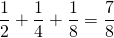We need to work out what number we would multiply by this to get one – i.e. the whole job being done. In this case it works out as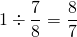which works out (a bit imprecisely) with a bit of work, or a decent calculator, as one hour, eight minutes and thirty four seconds and a bit. So, not great, but the second part of the puzzle works out much more smoothly. If we keep on with out pattern, we get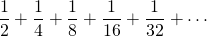Formally, this is called a Geometric Progression, there is a constant factor between each terms. Sometimes these infinite sums actually have a finite answer, which might be surprising. If you keep adding these fractions you might see that are adding ever closer to 1. Therefore this potentially infinite number of counters gets the work done in one hour, it can’t be less.

So, I was happy the second number worked out nicely, but the first is pretty tricky, and not easily worked out in one’s head. So I wondered what numbers I could use instead that would work out as an exact number of minutes. This really means that my three fractions from the first part of the problem, divide perfectly into 60. I used some Python to help me with this with a list comprehension:

It turns out that restricting ourselves to three numbers for the first quiz all of which are under 100 that there are 902 such sets of numbers. The very smallest numbers are 1, 2 and 6. The problem is that most of these triples don’t have the property of my original choices – which that there is a common number multiplied between the first and second, and the second and third etc.. That would make the second part of the puzzle more difficult.

So, I modified my list comprehension a bit to add the condition that there was a common ratio from a to b to c:

This produced just three triples (with all numbers under 100):

and you can see these are all quite related. So I grabbed the first three numbers to try and keep the puzzle small.

As well as that, the programme team wanted a different focus than an election – they were a bit worried that because it was in the news so much it would be better to have another focus. I considered a computational task divided between processors, but eventually concluded this wouldn’t make a lot of sense to some listeners, so I went with this final configuration of the puzzle.

Part One

A Professor gives her team of three PhD students many calculations to perform. The first student is the most experienced and can complete the job on her own in 7 hours, the next would take 14 hours on his own, and the last would take 28 hours working single handed to complete the task. How long would the task take if they all worked together?

Part Two

If the Professor has more helpers, but which follow the same pattern of numbers to complete the task, what is the absolute minimum time the task can take?

You can probably answer this from the details of the construction above, but if not, you can always cheat here (the BBC programme page) or here.

# My Puzzle for the Day

In November 2018 the BBC Radio 4 Today Programme was visiting Ulster University for an outside broadcast. I was asked to write the Puzzle for the Day for the broadcast. Here is my puzzle and some discussion about how it can be solved. The puzzle and a very brief solution is on the BBC page, but I restate it below, together with a more full solution.

Part One

A Professor gives her team of three PhD students many calculations to perform. The first student is the most experienced and can complete the job on her own in 7 hours, the next would take 14 hours on his own, and the last would take 28 hours working single handed to complete the task. How long would the task take if they all worked together?

Part Two

If the Professor has more helpers, but which follow the same pattern of numbers to complete the task, what is the absolute minimum time the task can take?

### Solving Part One.

Possibly the easiest way to solve the first part is to consider how much work can be done by each team member in a single hour and adding to get the total amount of work done per hour. For instance, the first team member can do the whole job in seven hours, so they can doof the job in one hour etc. So the team together can do the following in one hour: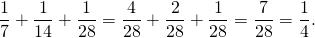In other words, one quarter of the job can be done in a single hour by the team of three working together. It follows that to do the whole job, the team needs four hours.

### Solving Part Two.

So what about part two of the puzzle? Quite reasonably, some people attempting the puzzle assumed that the more and more team members we would add, the time to complete the task would eventually drop to zero. This seems fairly intuitive – if you have potentially infinite team members then the total time must drop to zero.

But imagine we continue our pattern from above, for far more than three team members. This would be the proportion of work done in one hour by even an infinite team.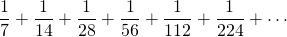If this addition produces an infinite “answer” then an infinite work rate per hour would certainly suggest the task could be done instantly. Surprisingly perhaps this sum of infinitely many numbers does not have an infinite answer. It may be a little easier to see its nature if we take out a factor of.

Then the summation looks like this: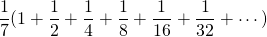It may be intuitive for some people that the numbers inside the bracket will get over closer to 2 – setting aside the initial 1, each subsequent number added goes half way from the previous sum to adding an additional 1 in total. In other words, this whole sum will be.

Some infinite summations do indeed have finite answers, but it can be quite difficult to prove which are which, or to find the summations if they are finite. However, this example, aside from intuitively having a relatively easy answer falls into a special category of such summations called a geometric progression. These are series of the form: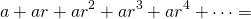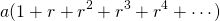In other words, each item in the sum is the previous item multiplied by some common ratio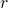. There is a nice formula for the sum of the firstterms of such progressions which you could use to solve part one – though it would rather be a sledgehammer to crack a nutshell, but there is a formula for the infinite summation too.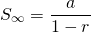(provided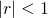, or in other words thatis between -1 and 1 not-inclusive, if not then the summation is infinite).

In this case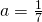andandpasses the test above so the infinite sum is indeed verified to be.

Finally therefore, if even infinitely many team members can only doof the job in an hour, we need to work out the number to multiply on this to get to the whole job being done, i.e. not “2/7” of the job but the whole “1” of the job.

That number is, so the whole job can be reduced from the four hours of part one to not less than 3.5 hours.

Of course, there are other ways to solve the puzzle. This is just one example pathway. If you are interested in how I went about constructing the puzzle, I detail that in another post.

Incidentally, the fact that summations of infinitely many objects sometimes has finite answers is of vital importance for many real life applications of mathematics. The whole subject of Integral Calculus relies on this, and for instance, this is closely related to why it is possible, with finite energy for a rocket to escape Earth or an electron to escape an atom.

# Infinity, A Very Short Introduction, by Ian Stewart

This review was originally written for the London Mathematical Society November 2018 Newsletter. The book can be found here.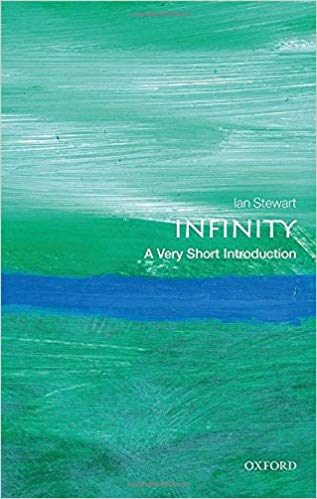The “Very Short Introduction” series by Oxford University Press attempt to take a moderately deep dive into various subjects in a slimline volume. Professor Stuart addresses the apparent paradox of tackling the subject of the infinite in such a small volume right at the start, along with the observation that the topic of infinity has long provided such paradoxes. This particular VSI aims to tackle infinity as found in numbers, geometry, art, theology, philosophy and more, and so it is a tightly packed volume indeed.

Infinity is a concept that is, at least now, embraced in Mathematics, but also reaches into Physics, Philosophy, Theology and Language in significant measure. In this book, Ian Stewart sets off almost immediately into the mathematical interpretations and concepts of infinity, starting with examples that are likely to be accessible to a wide range of readers, but also touching on some that will cause more mathematically advanced readers to consider them carefully and which may be challenging to less mathematically literate readers.

These examples are of the paradoxical issues surrounding infinity; all but one of these is explicitly mathematical, some geometrical, others more algebraic; by the end of the first short chapter we have visited David Hilbert’s famous hotel and explored some of the implications for the arithmetic of the infinite.

The second chapter then moves into a more detailed exploration of the consequences of the infinite in numbers, and in particular explores the infinite, non-repeating decimal representations of irrational numbers and the continuity of the real numbers.

Stewart then explores the history of the infinite in the third chapter, and how it weaved through early Greek philosophy and the classic paradoxes of Zeno, and how for the Greeks issues of infinity were closely tied to their thoughts and theories about motion, and indeed whether motion was in fact possible or an illusion. Some time is spent with Aristotle, and how he dismissed the idea of an “actual” infinity in favour of a “potential” infinity. We then move through with both Locke and Kant to the beginning of more modern philosophical analyses of the infinite. Some time is taken to explore the philosophy of the infinite in Christian theology, particularly through Thomas Aquinas, a philosopher heavily influenced by Aristotle and how he used the infinite in his “proof” of God.

Stewart also explores how, in the modern era, mathematicians take the infinite very much as a normal and integral part of mathematics, with little concern about the distinction of actual and potential infinities that were the great concern of the philosophy of the ancient world.

We dive then, from the infinitely large to the infinitely small in the fourth chapter where the seeds of calculus and analysis are to be seen, and the philosophical objections from Bishop Berkeley to the use of infinitesimals. It is interesting to note that these and other concerns about the theoretical underpinnings of calculus were largely ignored in the face of its obvious utility, until others tried to explore these foundations more deeply. Stewart takes us through this work through Cauchy and eventually to the work of Bolzano and Weierstrass who finally introduced the ? and ? notation that has undoubtedly delighted many undergraduates since and ushered in the start of analysis proper. Stewart than dips into an examination of non-standard analysis, a topic that at least I was never knowingly exposed to in my formal studies; it was intriguing to read of these numbers with “standard” and “infinitesimal” parts.

There follows a chapter on the geometrically infinite, which in particular looks at the role of the infinite in art, but which again after an informal discussion dips into the mathematics of what is going on. The chapter after this focuses on infinities that arise in Physics, particularly in optics, Newtonian and Relativistic gravity, moving on then to discuss the size of the known universe and its curvature. These two chapters are both short and may require some unpacking by readers with less background knowledge.

The final chapter is mostly dedicated to work of Cantor and his systemization of modern mathematical thinking around the concept of the infinite. Here we meet the distinctions between the finite, countably infinite and uncountably infinite, transfinite cardinals and transfinite ordinals. But even here we find the objections of some philosophers, in this case Wittgenstein. This is interesting to read in an era where Cantor’s formulations are considered uncontroversial and part and parcel of the “paradise” of Hilbert’s modern mathematics in the same way that the past controversies of complex numbers are of little interest to modern mathematicians.

The approach taken to infinity in the book, is non-apologetically Pure Mathematical in its spirit, and I suppose this may make the work a little less accessible for some readers, particularly those who are not prepared to think through some of the sections, perhaps with a pen and paper. The Very Short Introduction to Mathematics, from the same series, by Timothy Gowers similarly tackles a cross section of challenging examples from the discipline in a relatively small space.

In September 2016, the BBC aired an interesting series on Radio 4: “The History of the Infinite” (this is still happily available online for those interested, at least for those in the UK). In this series, Adrian Moore began discussing the original Greek antipathy to the idea in early philosophy, and then how the idea emerged through Aristotelian Philosophy, Christian theology. It was after this that Moore decided to tackle the more serious implications of the infinitely small and big in mathematics, before emerging back through Physics into more philosophical territory.

I suspect this route, sandwiching the more complicated mathematical treatment between philosophy more related to human experience could be more palatable to a general reader.

The Very Short Introduction to Infinity is nevertheless a fascinating and joyful exploration of the topic, accessible to the committed and careful novice, but with enough detail and asides to delight formally mathematically trained readers.